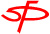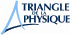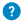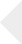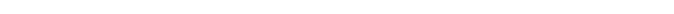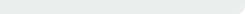The Meissner effect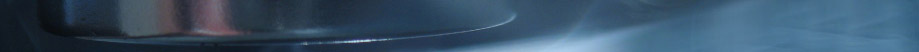# The Meissner effect

## Magnetic fields expelled

This Meissner effect happens when electric current loops spontaneously appear on the surface of a material that becomes superconducting in the presence of a magnetic field. These currents create a magnetic field, similar to that of an electromagnet. These currents adjust to exactly compensate the magnetic field applied inside the superconductor. The total magnetic field hence becomes equal to zero in the sample volume, and the superconductor is protected, except on its surface where the currents develop. Thanks to these supercurrents, the superconductor expels the magnetic field. Since there is no electric resistance in a superconductor, these currents can exist forever without consuming any energy.

When the magnetic field in the sample is caused by a magnet, the magnetic field created by these supercurrents applies a force on the magnet and expels it. The magnet will then start to levitate at a distance that marks the balance between the repulsive force and the weight of the magnet attracted by gravity.

When the magnetic field in the sample is caused by a magnet, the magnetic field created by these supercurrents applies a force on the magnet and expels it. The magnet will then start to levitate at a distance that marks the balance between the repulsive force and the weight of the magnet attracted by gravity.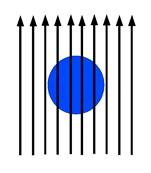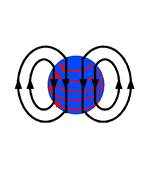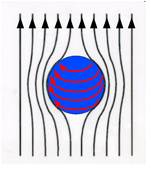a) The black arrows represent the external magnetic field applied to the superconducting sample.
b) Currents appear on the surface of the superconductor (in red). These currents create a magnetic field (the external magnetic field is not represented).
c) The total magnetic field is the sum of the applied magnetic field and the magnetic field that appears as a result of the reaction. In the superconductor, as a whole, the magnetic field is equal to zero.

This experiment was conducted for the first time in 1947 with lead, which becomes superconducting below 7,2 K [http://www.nature.com/physics/looking-back/arkadiev].